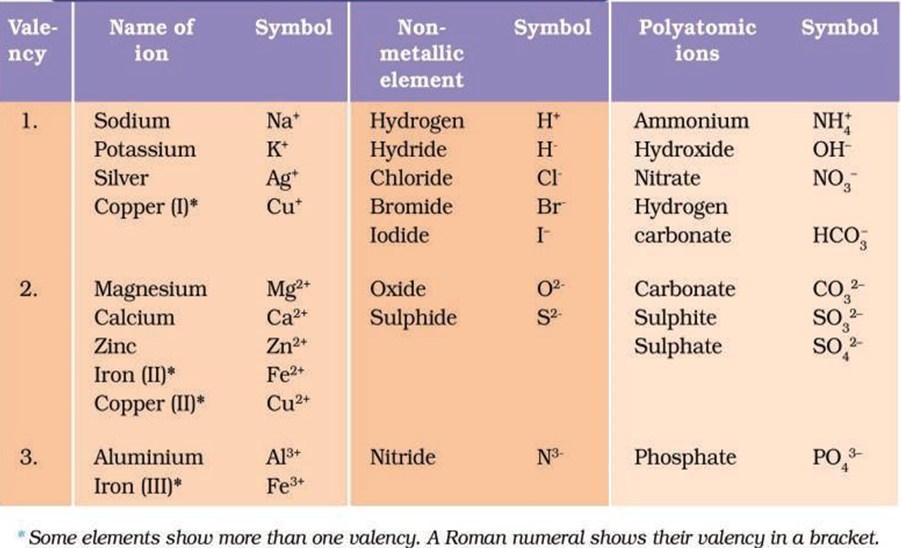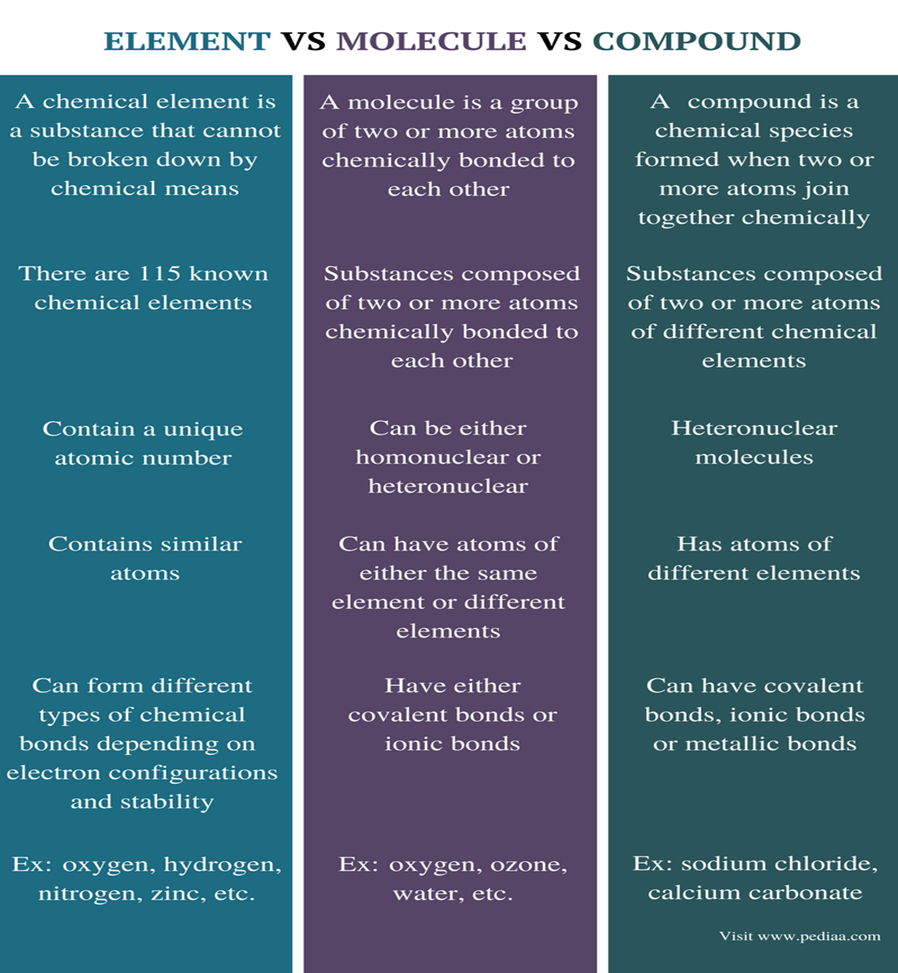top of page

# Atoms and Molecules - Class 9 Science Notes## Laws of Chemical Combination

Antoine L. Lavoisier laid the foundation of chemical sciences by establishing two important Laws of Chemical Combinations, which are:

(i) Law of Conservation of Mass

(ii)Law of Constant Proportions

### Law of Conservation of Mass

The law of conservation of mass states the “Mass can neither be created nor be destroyed in a Chemical reaction”. During a chemical reaction, the sum of the masses of the reactant and products remains unchanged.

### Law of Constant Proportions

Lavoisier noted that many compounds were composed of two or more elements and each such compound had the same elements in the same proportions irrespective of where the compound came from or who prepared it.

For example, in compound such as water, the ratio of the mass of hydrogen to the mass of the oxygen is always 1:8, whatever the source of water. Thus, if 9 gm of water is decomposed, 2 gm of hydrogen and 8 gm of oxygen are always obtained.

Similarly in ammonia, nitrogen and hydrogen are always present in the ratio of 14:3 by mass.

This led to the law of constant proportions which is also known as law of definite proportions.

Proust also stated the law as “In a chemical substance the elements are always present in definite proportions by mass”.

### Dalton’s Atomic Theory:

According to this theory, all matter, whether an element, a compound or a mixture is composed of small particles called Atoms.

The postulates of the theory are as follows:

1. All matter is made up of very tiny particles called atoms, which participate in the chemical reactions.

2. Atoms are indivisible particles, which cannot be created or destroyed in a chemical reaction.

3. Atoms of a given element are identical in mass and chemical properties.

4. Atoms of different elements have different masses and chemical properties.

5. Atoms combine in the ratio of small whole number to form compounds.

6. The relative number and kinds of atoms are constant in a given compound.

An Indian philosopher, Maharishi Kanad, postulated that if we go on dividing matter (padarth), we shall get smaller and smaller particles and ultimately come cross the smallest particle beyond which further division will not be possible. He named these particles as Parmanu.
Pakudha Katyayama, an Indian philosopher stated that these particles (smallest particle of matter) normally exist in a combined form which gives us various forms of matter.
Democritus, an ancient Greek philosopher, suggested that if we go on dividing matter, a stage will come when particles obtained cannot be divided further, he called these indivisible particles as atoms.

### What is an Atom?

An atom is the smallest particle of an element that cannot usually exist independently and retains all its chemical properties.

The building blocks of all the matter are atoms.

Atoms are very small. More than millions of atoms when stacked would make a layer barely as thick as sheet paper.What are the modern-day symbols of atoms of different elements?• Dalton was the first to use the symbols for Elements in a very specific sense.

• Berzilius suggested that the symbols of elements be made from one or two letters of the name of the element.

• In the beginning, the name of the elements was derived from the name of the place where they were found for the first time.

For example, the name Copper was taken from Cyprus.

• Some names were taken from specific colours. For example, Gold from English word meaning Yellow.

• Now-a-days IUPAC (International Union for Pure and Applied Chemistry) approves names of elements, symbols and units.

1. Many symbols are the first one or two letters of the element’s name in English.

The first letter of symbol is always capital letter (uppercase) and the second the letter as a small letter (lowercase).

For example:

(a) hydrogen H

(b) aluminium Al (not AL)

(c) cobalt Co (not CO)

2. Symbols of some elements are formed from the first letter of the name and a letter appearing later in the name.

For example:

(a) chlorine Cl

(b) zinc Zn

3. Other symbols have been taken from the names of the elements in Latin, German and Greek.

For example: (a) the symbol of iron is Fe from its Latin name ferrous.

(b) sodium is Na from Natrium.

(c) potassium is K from kalium.

Each element has a name and a unique chemical symbol.

#### Symbols for some Elements

 Element Symbol Element Symbol Element Symbol Aluminium Al Copper Cu Nitrogen N Argon Ar Fluorine F Oxygen O Barium Ba Gold Au Potassium K Boron B Hydrogen H Silicon Si Bromine Br Iodine I Silver Ag Calcium Ca Iron Fe Sodium Na Carbon C Lead Pb Sulphur S Chlorine Cl Magnesium Mg Uranium U Cobalt Co Neon Ne Zinc Zn

#### What is Atomic Mass?

According to Dalton’s Atomic theory, each element had a characteristic atomic mass.

Initially, scientist took 1/16th of the mass of an oxygen atom as the unit. This was considered relevant for two reasons:

1. Oxygen reacted with a large number of elements and formed compounds.

2. This atomic mass unit gave masses of most of the elements as whole numbers.

However, in 1961 a universally accepted atomic mass unit, carbon-12 isotope was chosen as the standard reference for measuring atomic masses.

One atomic mass unit is a mass equal to exactly one-twelfth (1/12th) of the mass of one atom of carbon-12

Earlier atomic mass unit was designated as “amu” but it is designated as “u”.

#### Atomic masses of a few elements

 Element Atomic mass (u) Hydrogen 1 Carbon 12 Nitrogen 14 Oxygen 16 Sodium 23 Magnesium 24 Sulphur 32 Chlorine 35.5 Calcium 40

Similarly, the relative atomic mass of the atom of an element is defined as the average mass of the atom, as compared to 1/12th the mass of one carbon-12 atom.

#### How do Atoms exist?

Atoms of most elements are not able to exist independently. Atoms form molecules and ions. These molecules or ions aggregate in large number to form the matter that we can see, feel or touch.

#### What is a Molecule?

• A molecule is in general a group of two or more atoms that are chemically bonded together, that is, tightly held together by attractive forces.

• A molecule can be defined as the smallest particle of an elements or a compound that is capable of an independent existence and shows all the properties of that substance.

• Atoms of the same elements or a different element can join together to form molecules.

Difference between the Molecules of Elements and Molecules of Compounds.

Molecule of Elements Molecule of Compounds

 Definition A combination of two or more atoms of same type. A combination of two or more atoms of different type. Chemical Elements Has atoms of one chemical element. Has atoms of two or more chemical elements. Chemical Bonding Has covalent bond. Have Their covalent or ionic bond. Examples O2, Cl2, H2, Br2, etc. H2O, NH3, SO3, etc

#### Atomicity of Some ElementsMolecules of some compounds### What is an Ion?

• Compounds composed of metals or non-metals contains charged species. These species are known as ions.

• It may consist of a single charged atom or a group of atoms that have a net charge on them.

• An ion can be negatively or positively charged species.• A negatively charged ion is called an ‘anion’. For example, Cl-, SO43-, etc. and the positively charged ion is called a ‘cation’. For example, Na+, Al3+, etc.

• In, Sodium Chloride (NaCl), its constituent particles are positively charged sodium ion (Na+) and negatively charged chloride ion (Cl-).

• Single species carrying a charge is known as monoatomic ion, for example; Cu+ etc. whereas a group of atoms carrying a charge is known as polyatomic ion, for example; NO3- etc.

Names and Symbols of some ions### Writing Chemical Formulae

The chemical formula of a compound is a symbolic representation of its composition. (or A chemical formula of compound shows its constituent elements and the number of the atoms of each combing elements)

• To write chemical formula we need to know the symbols and combining capacity of the elements.

The chemical formula of a molecular compound is determined by the valency of each element.

The combining power (or capacity) of an element is known as its valency.

The rules to be followed while writing a chemical formula:

1. The valencies or charges on the ion must balance.

2. When a compound consists of a metal and a non-metal, the name or symbol or the metal is written first (or left). For example: calcium oxide (CaO), sodium chloride (NaCl), iron sulphide (FeS), copper oxide (CuO)etc., where oxygen, chlorine, sulphur are non-metals and are written on the right.

3. In compounds formed with polyatomic ions, the number of ions present in the compound is indicated by enclosing the formula of ion in a bracket and writing the number of ions outside the bracket. For example: Mg(OH)2.

In case the number of polyatomic ions is one the bracket is not required. For example, NaOH.

#### Formulae of Simple Compound:

The simplest compounds which are made up of two different elements are called binary compounds.

- While writing the chemical formulae for compounds, we write the constituent elements and their valencies as shown below.

- Then we must crossover the valencies of the combining atoms.Some more examples:### Molecular Mass and Mole Concept

Molecular Mass: The molecular mass of a substance is the sum of the atomic masses of all the atoms in a molecule of the substance.

Formula Unit Mass: The formula unit mass of a substance is a sum of the atomic masses of all atoms in a formula unit of a compound.

The only difference in formula unit mass from molecular mass is that, we use it for those substances whose constituent particles are ions.

Mole Concept:

2H2 + O2 -> 2H2O

The above reaction indicates that

(i) Two molecules of hydrogen combine with one molecule of oxygen to form two molecules of water, or

(ii) 4 u of hydrogen molecules combine with 32 u of oxygen molecules to form 36 u of water molecules.

It is more, convenient to refer to the quantity of a substance in terms of the number of its molecules or atoms, rather than their masses.

One mole of any species (atoms, molecules, ions or particles) is that quantity in number having a mass equal to its atomic or molecular mass in grams.

The word mole was introduced by Wilhelm Ostwald in 1896

The number of particles (atoms, molecules, ions) present in 1 mole of any substance is fixed, with a value of 6.022 * 1023. This is known as Avogadro Number or Avogadro Constant (N0) and is an experimentally value.

1 mole (of anything) = 6.022 * 1023 in number

The mass of 1 mole of a substance is equal to its relative atomic or molecular mass in grams.

Molar mass of atoms is also known as gram atomic mass

Atomic mass of hydrogen = Gram atomic mass of hydrogen

1 u = 1 g

1 u (atomic unit) hydrogen has only 1 atom of hydrogen

1 g hydrogen has 1 mole atoms = 6.022 * 1023 atoms of hydrogen

Similarly,

16 u oxygen = 1 atom of oxygen

16 g oxygen = 1 mole of atom = 6.022 * 1023 atoms of oxygenSee All
bottom of page